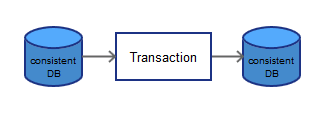# ML Wiki

## Consistency

For databases, consistency means satisfying integrity constraints, which are about the correctness of the data in a database. So a database is consistent if all its constraint are satisfied.

### Integrity Constraints

Some of the integrity constraints are:

• entity integrity constraint (a primary key cannot be null)
• referential integrity constraint (if a tuple $X$ in one relation refers to some other tuple $Y$ in another relation, $Y$ must always exist in that relation)

Examples of predicates that must hold:

• $x$ is a key of relation $R$
• Functional Dependency $x \to y$ holds in $R$
• domain($x$) = {Red, Green, Blue} - the only allowed values
• no employee should make more than twice average salary (achieved with triggers in Active Databases)

In a database to specify if data is valid we use constraints.

## Transaction Constraints

Transaction Consistency

• essentially involve two database states: the old state (before $T$) and the new state (after $T$)
• but always maintaining a database in a consistent state is impossible

Example:

• we have $n$ accounts in a bank: $a_1, ..., a_n$
• suppose that we store the total sum somewhere in the database
• constraint: $a_1 + ... + a_n = \text{TOTAL}$
• but during a transaction the database may be in inconsistent state
• transaction: deposit 100 USD to $a_2$
• to do that we need:
• update $a_2: a_2 \leftarrow a_2 + 100$
• (at this moment the constraint is not satisfied)
• update TOTAL: $\text{TOTAL} \leftarrow \text{TOTAL} + 100$
• so during the transaction we'll have a state in which the DB is not consistent

We can define a transaction as a sequence of updates on the database.

• It preserves consistency if executing it brings a database from one consistent state to another.
• The database doesn't have to be consistent during the transaction.
• For transactions, consistency is the letter "C" in the ACID.
•• And a transaction should happen in Isolation (Letter "I" in ACID)

### Crash Recovery

But what if during the execution of a transaction a crash occurs?

## Consistency Models

For Distributed Databases maintaining consistency is harder. Consistency models determine rules for visibility and order of updates.

### Strict Consistency

• every replica sees every update in the same order
• all reads return the most up-to-date data no matter what replica is asked
• need to employ some techniques for commit propagation, for example, Two-Phase Commit
• according to the CAP Theorem, cannot achieve strict consistency at the same time with partition-tolerance

### Eventual Consistency

• as $t \to \infty$ all readers will see the writes
• but updates are not atomic as in case of Strict Consistency

### Weak Consistency

• every replica will see updates
• but there's no guarantee on the order

in this case later updates may be overwritten by earlier ones because they arrived later